# Examples#

Examples of several functionalities of the package.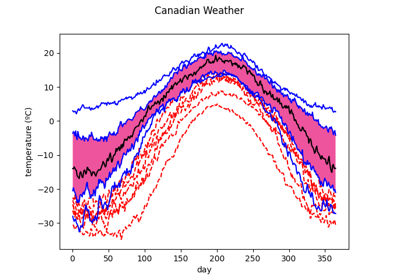Boxplot

BoxplotClassification methods

Classification methods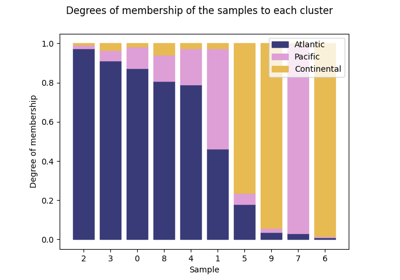Clustering

Clustering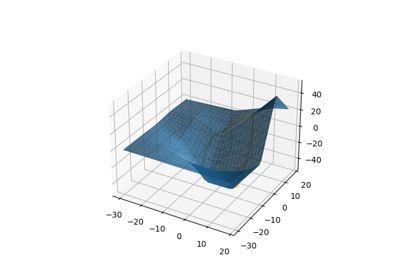Function composition

Function composition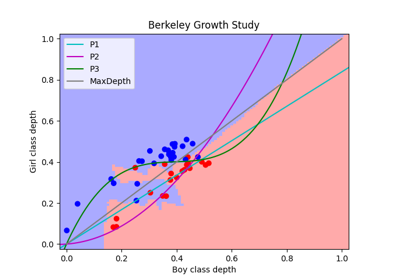Depth based classification

Depth based classification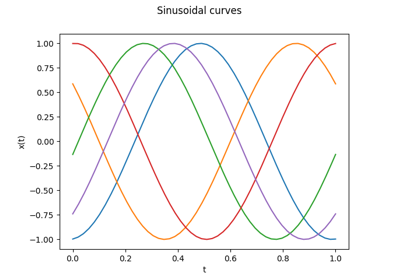Discretized function representation

Discretized function representation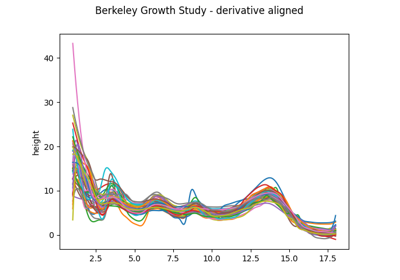Elastic registration

Elastic registration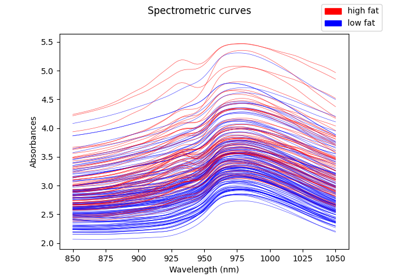Exploring data

Exploring data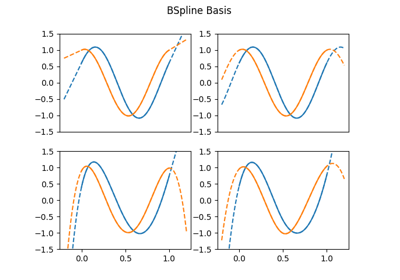Extrapolation

Extrapolation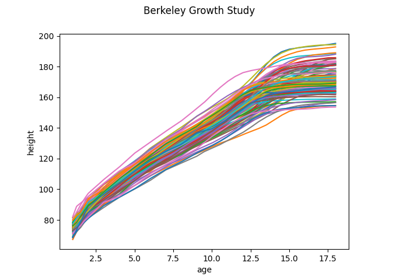Functional Principal Component Analysis

Functional Principal Component Analysis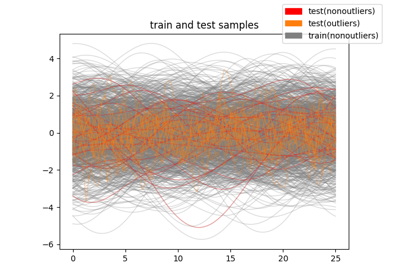Outlier detection with FPCA

Outlier detection with FPCA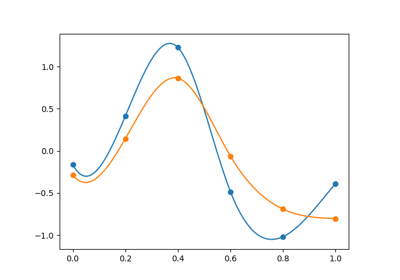Interpolation

Interpolation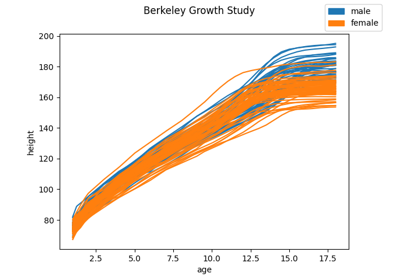K-nearest neighbors classification

K-nearest neighbors classification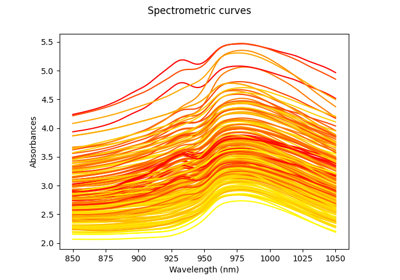Kernel Regression

Kernel Regression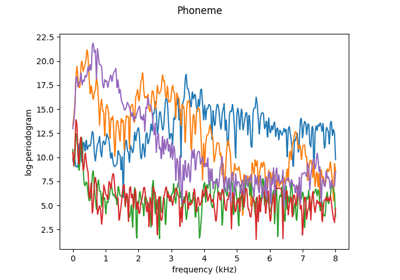Kernel Smoothing

Kernel Smoothing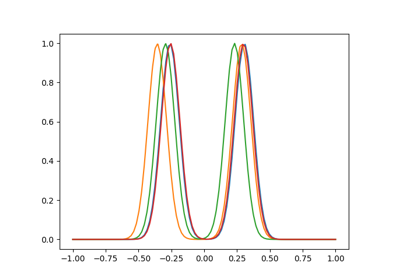Landmark registration

Landmark registration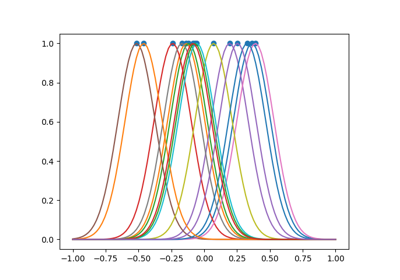Landmark shift

Landmark shift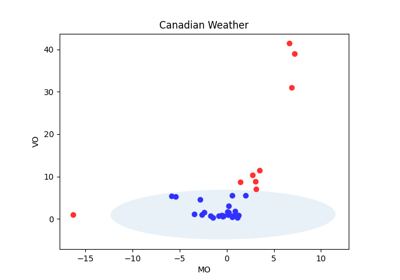Magnitude-Shape Plot

Magnitude-Shape Plot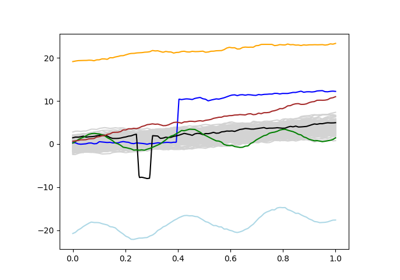Magnitude-Shape Plot synthetic example

Magnitude-Shape Plot synthetic example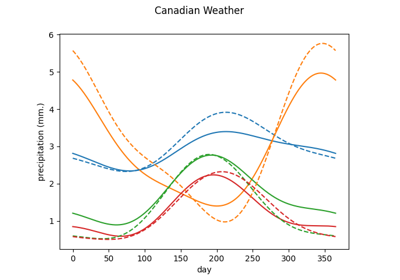Neighbors Functional Regression

Neighbors Functional Regression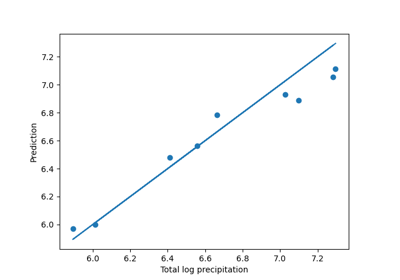Neighbors Scalar Regression

Neighbors Scalar Regression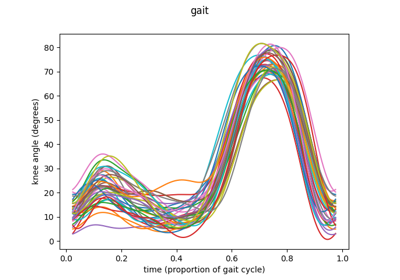One-way functional ANOVA with real data

One-way functional ANOVA with real data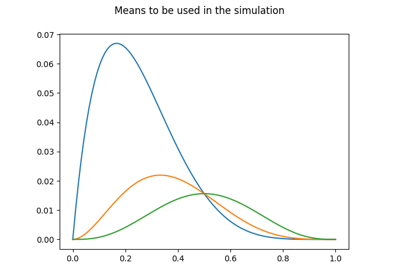One-way functional ANOVA with synthetic data

One-way functional ANOVA with synthetic data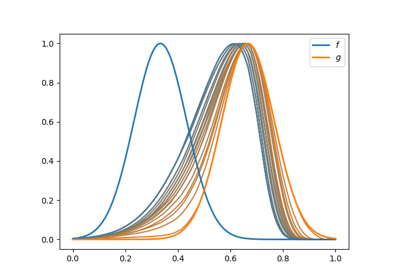Pairwise alignment

Pairwise alignment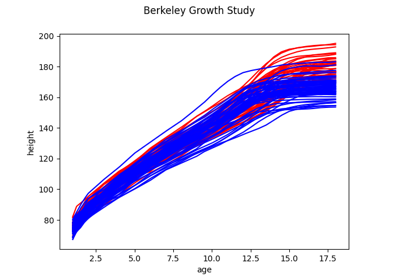Representation of functional data

Representation of functional dataShift Registration

Shift Registration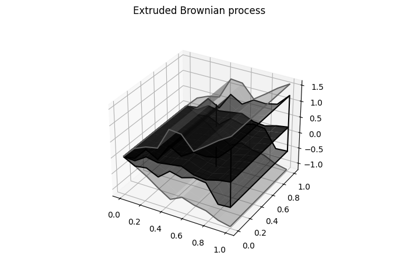Surface Boxplot

Surface Boxplot

# ICTAI examples#

Examples of complete analyses showcasing the main functionalities of the scikit-fda package, presented at the 34th IEEE International Conference on Tools with Artificial Intelligence (ICTAI).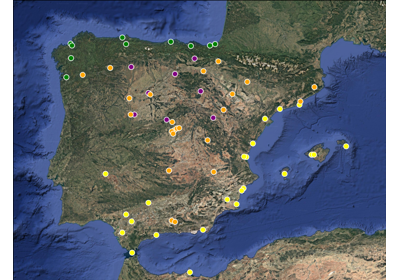Meteorological data: data visualization, clustering, and functional PCA

Meteorological data: data visualization, clustering, and functional PCA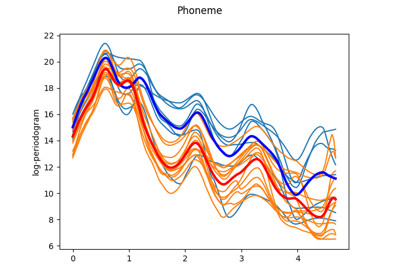Voice signals: smoothing, registration, and classification

Voice signals: smoothing, registration, and classification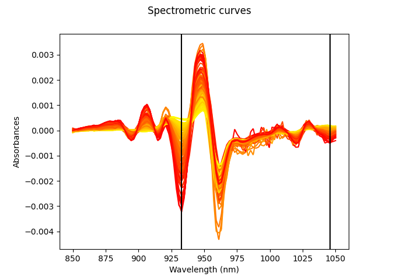Spectrometric data: derivatives, regression, and variable selection

Spectrometric data: derivatives, regression, and variable selection

Gallery generated by Sphinx-Gallery